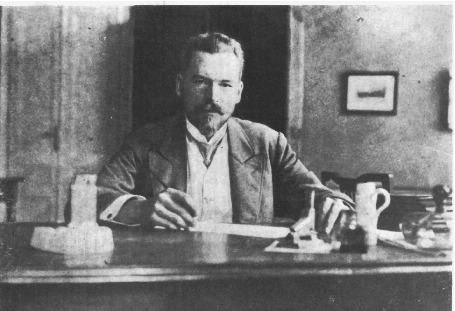#31st Marian Smoluchowski Symposium on Statistical Physics

2-7 September 2018
Europe/Warsaw timezone

## Quantum law for partition of kinetic energy

3 Sep 2018, 14:30
45m
talk

### Speaker

Prof. Jerzy Luczka (University of Silesia)

### Description

One of the fundamental laws of classical statistical physics is the energy equipartition theorem which states that for each degree of freedom the average kinetic energy equals $E_k=k_B T/2$, where $k_B$ is the Boltzmann constant and $T$ is temperature of the system. Despite the fact that quantum mechanics has already been developed for more than 100 years still there is no quantum counterpart of this theorem. We attempt to fill this far-reaching gap and formulate the \emph{quantum law for equipartition of energy} in the appealing form $E_k = \langle \mathcal E_k \rangle$, where $\mathcal E_k$ is thermal kinetic energy per one degree of freedom of the thermostat consisting of harmonic oscillators and $\langle ...\rangle$ denotes averaging over frequencies $\omega$ of those thermostat oscillators which contribute to $E_k$ according to the probability distribution $\mathbb P(\omega)$. %It is valid for an arbitrary strength of the system-thermostat coupling. We derive it for two paradigmatic and exactly solvable models of quantum open systems: a free Brownian particle and a harmonic oscillator. We formulate conditions for validity of the relation $E_k = \langle \mathcal E_k \rangle$ for other quantum systems.

### Primary author

Prof. Jerzy Luczka (University of Silesia)

### Co-authors

Dr. Jakub Spiechowicz (University of Silesia) Mr. Paweł Bialas (University of Silesia)

### Presentation Materials

There are no materials yet.
###### Your browser is out of date!

Update your browser to view this website correctly. Update my browser now

×